﻿ 用于脑机接口的训练样本集扩增方法 Amplification Method of Training Sample Set for Brain Computer Interface

Computer Science and Application
Vol. 09  No. 02 ( 2019 ), Article ID: 28758 , 12 pages
10.12677/CSA.2019.92027

Amplification Method of Training Sample Set for Brain Computer Interface

Yuanzi Liu, Yan Wu, Zhaohua Lu, Qi Li*

School of Computer Science and Technology, Changchun University of Science and Technology, Changchun Jilin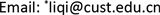Received: Jan. 15th, 2019; accepted: Jan. 25th, 2019; published: Feb. 1st, 2019ABSTRACT

In order to improve the classification accuracy of EEG signals, a method based on sample capacity amplification was proposed in this research of brain-computer interface technology. This method doubled all the electrode data value in one session of EEG data and then trained them as samples together with the original data, and this method not only increased the sample capacity, but also further improved the convergence speed of the train and the reliability of the test. After amplifying the public sample capacity, the new data and the original data were trained and tested by Bayesian Linear Discriminant Analysis method under the paradigm of row and column flash of brain-computer interface, then data were projected on a straight line in two categories of data. The results showed that the projected points of each kind of new data after projection were closer than the original public data, the center points of the two categories were larger and also the classification effect was better than the original public sample data and the average accuracy has been significantly improved compared with the average accuracy of the original public data. These results reflected that this amplification method of training sample set for brain computer interface can significantly improve the classification accuracy of EEG signals. An example was given to verify the validity of the proposed sample amplification method, which provided a feasible solution to the problem of insufficient sample capacity.

Keywords:Brain-Computer Interface, Sample Capacity, Bayesian Linear Discriminant AnalysisCopyright © 2019 by author(s) and Hans Publishers Inc.

This work is licensed under the Creative Commons Attribution International License (CC BY).

http://creativecommons.org/licenses/by/4.0/1. 引言

2. 方法

2.1. 实验一

2.1.1. 行列闪范式Figure 1. The paradigm of row and column flash

2.1.2. 样本容量扩增Table 1. The comparison of data changes in the first ten sampling points on the Pz electrodeTable 2. The comparison of the number of samples before and after amplification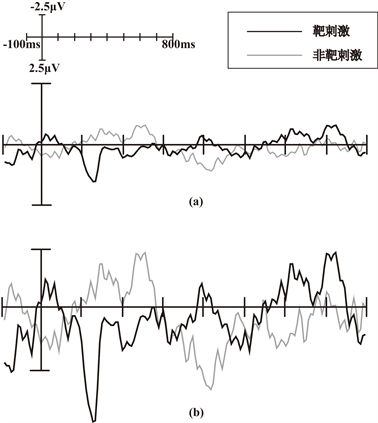Figure 2. The comparison of target and non target stimulus waveform in Pz electrode before and after the sample data changed. (a) T he comparison of target and non target stimulus waveform before the sample data changed; (b) The comparison of target and non target stimulus waveform after the sample data changed

2.2. 实验二

2.2.1. 单闪实验范式

2.2.2. 样本容量扩增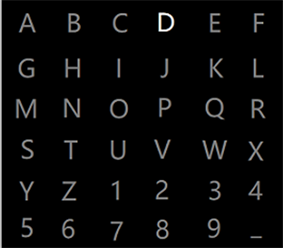Figure 3. The paradigm of single flashTable 3. The comparison of the number of samples before and after amplification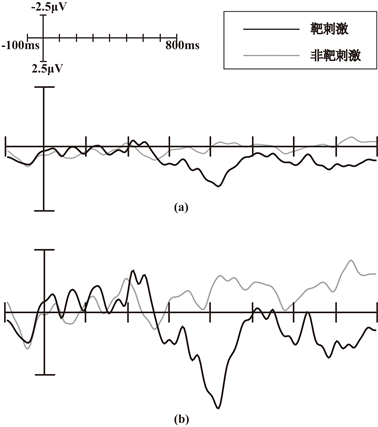Figure 4. The comparison of target and non target stimulus waveform in Pz electrode before and after the sample data changed. (a) The comparison of target and non target stimulus waveform before the sample data changed; (b) The comparison of target and non target stimulus waveform after the sample data changed

3. BLDA分类

3.1. BLDA分类算法

3.1.1. 线性最小二乘回归

$J\left(\omega \right)=\underset{i=1}{\overset{n}{\sum }}{\left({y}_{i}-{\omega }^{\text{T}}{x}_{i}\right)}^{2}$ (1)

$\omega ={\left(X{X}^{\text{T}}\right)}^{-1}Xy$ (2)

$J\left(\omega \right)=\underset{i=1}{\overset{n}{\sum }}{\left({y}_{i}-{\omega }^{\text{T}}{x}_{i}\right)}^{2}+\lambda {\omega }^{\text{T}}{I}^{\prime }\omega$ (3)

$\omega ={\left(X{X}^{\text{T}}+\lambda {I}^{\prime }\right)}^{-1}Xy$ (4)

${y}_{i}={\omega }^{\text{T}}{x}_{i}$ (5)

3.1.2. BLDA分类算法

$y={\omega }^{\text{T}}x+n$ (6)

$p\left(\omega \right)=\frac{1}{a}\mathrm{exp}\left(-J\left(\omega \right)\right)$ (7)

$p\left(\omega \right)=\frac{1}{a}\mathrm{exp}\left(-\underset{i=1}{\overset{n}{\sum }}{\left({y}_{i}-{\omega }^{T}{x}_{i}\right)}^{2}\right)\mathrm{exp}\left(-\lambda {\omega }^{\text{T}}{I}^{\prime }\omega \right)$ (8)

$p\left(t|\beta ,\omega \right)={\left(\frac{\beta }{\text{2π}}\right)}^{\frac{n}{2}}\mathrm{exp}\left(-\frac{\beta }{2}{‖{X}^{\text{T}}\omega -y‖}^{2}\right)$ (9)

$p\left(\omega |\alpha \right)={\left(\frac{\alpha }{\text{2π}}\right)}^{\frac{t}{2}}{\left(\frac{\epsilon }{\text{2π}}\right)}^{\frac{1}{2}}\mathrm{exp}\left(-\frac{1}{2}{\omega }^{\text{T}}{I}^{\prime }\left(\alpha \right)\omega \right)$ (10)

$P\left(\omega |\beta ,\alpha ,t\right)=\frac{p\left(t|\beta ,\omega \right)P\left(\omega |\alpha \right)}{\int P\left(t|\beta ,\omega \right)P\left(\omega |\alpha \right)\text{d}\omega }$ (11)

$m=\beta \left(\beta X{X}^{\text{T}}+{I}^{\prime }\left(\alpha \right)\right)Xy,\text{\hspace{0.17em}}\text{\hspace{0.17em}}b={\left(\beta X{X}^{\text{T}}+{I}^{\prime }\left(\alpha \right)\right)}^{-1}$ (12)

$p\left(y|\beta ,\alpha ,x,t\right)=\int p\left(y|\beta ,x,\omega \right)P\left(\omega |\beta ,\alpha ,t\right)\text{d}\omega$ (13)

$\mu ={m}^{\text{T}}x,\text{\hspace{0.17em}}\text{\hspace{0.17em}}{\sigma }^{2}=\frac{1}{\beta }+{x}^{\text{T}}bx$ (14)

$\alpha =\frac{t}{\underset{i=1}{\overset{t}{\sum }}{c}_{ii}+{m}_{i}^{2}},\text{\hspace{0.17em}}\text{\hspace{0.17em}}\text{\hspace{0.17em}}\beta =\frac{n}{tr\left(X{X}^{\text{T}}b\right)+‖{X}^{\text{T}}m-y‖}$ (15)

3.1.3. BLDA对P300信号的识别

3.2. 实验一数据分类结果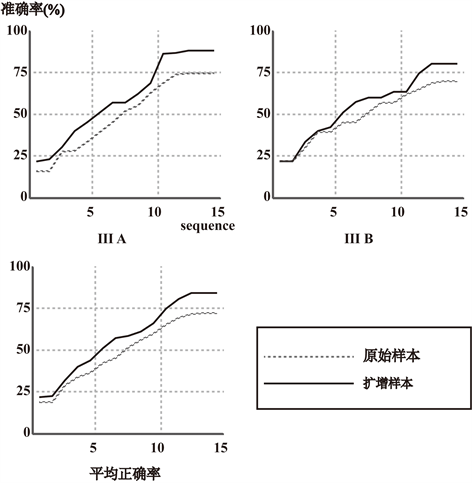Figure 5. The comparison of BLDA classification accuracy between original sample and amplified sample

3.3. 实验二数据分类结果Figure 6. The comparison of BLDA classification accuracy between original sample and amplified sample

4. 讨论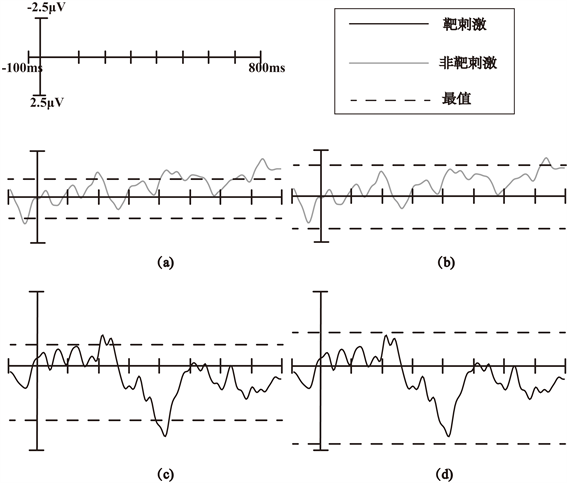Figure 7. The comparison of the maximum values obtained before and after sample trained in Pz electrode on test data. (a) Representation of the maximum value obtained from training original samples on the test data of non-target stimulus; (b) Representation of the maximum value obtained from training amplified samples on the test data of non-target stimulus; (c) Representation of the maximum value obtained from training original samples on the test data of target stimulus; (d) Representation of the maximum value obtained from training amplified samples on the test data of target stimulus

BLDA是一种有监督的学习方法，也就是说它的数据集每个样本都有类别的输出。在两种类别的数据中BLDA将数据在直线上进行投影，投影后每一种数据的投影点尽可能的接近，而两种类别的数据的类别中心点尽可能的大才会使得分类效果较好。如图8所示，我们把目标样本和非目标样本用灰色和黑色表示，用BLDA将两种类别数据投影到一条直线上。从直观上可以看出，右图比左图的投影效果要好，因为右图的黑色和灰色数据较为集中，且两种类别之间的距离也更明显。从BLDA原理来看，假设我们的数据集 $D=\left\{{x}_{1},{x}_{2},\cdots ,{x}_{m}\right\}$ ，其中任意样本xi为第i个数据。我们定义 ${X}_{j}\left(j=0,1\right)$ 是第j类样本的集合， ${N}_{j}\left(j=0,1\right)$ 是第j类样本的个数，而 ${\sum }_{j}\left(j=0,1\right)$ 是第j类样本的协方差矩阵， ${\mu }_{j}\left(j=0,1\right)$ 是第j类样本的均值，J(w)是BLDA投影到直线w后的损失函数。

μj的表达式为：

${\mu }_{j}=\frac{1}{{N}_{j}}\underset{x\in {X}_{j}}{\sum }x\left(j=0,1\right)$ (16)

j的表达式为：

${\sum }_{j}=\underset{x\in {X}_{j}}{\sum }{\left(x-{\mu }_{j}\right)}^{\text{T}}\left(j=0,1\right)$ (17)

J(w)的表达式为：

$J\left(w\right)=\frac{{|{\stackrel{¯}{\mu }}_{0}-{\stackrel{¯}{\mu }}_{1}|}^{2}}{{\stackrel{¯}{\sum }}_{0}^{2}+{\stackrel{¯}{\sum }}_{1}^{2}}$ (18)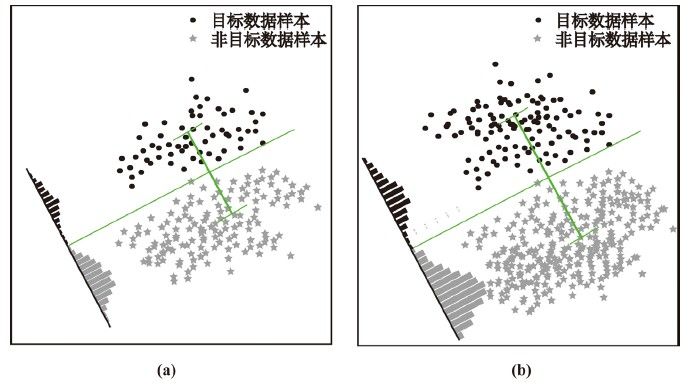Figure 8. The comparison of BLDA projection results of sample points before and after amplified in straight line. (a) BLDA projection results of original sample points in straight line; (b) BLDA projection results of amplified sample points in straight line

5. 结束语

Amplification Method of Training Sample Set for Brain Computer Interface[J]. 计算机科学与应用, 2019, 09(02): 227-238. https://doi.org/10.12677/CSA.2019.92027

1. 1. Hoffmann, U., Fimbel, E.J. and Keller, T. (2009) Brain-Computer Interface Based on High Frequency Steady-State Visual Evoked Potentials: A Feasibility Study. International IEEE/EMBS Conference on Neural Engineering, Antalya, 29 April-2 May 2009, 466-469. https://doi.org/10.1109/NER.2009.5109334

2. 2. Farwell, L.A. and Donchin, E. (1988) Talking Off the Top of Your Head: To-ward a Mental Prosthesis Utilizing Event-Related Brain Potentials. Electroencephalography & Clinical Neurophysiology, 70, 510. https://doi.org/10.1016/0013-4694(88)90149-6

3. 3. BCI Competition III Data. http://www.bbci.de/competition/iii/

4. 4. Mahanta, M.S., Aghaei, A.S. and Plataniotis, K.N. (2012) A Bayes Optimal Matrix-Variate LDA for Extraction of Spatio-Spectral Features from EEG Signals. International Conference of the IEEE Engineering in Medicine and Biology Society, 2012, 3955-3958. https://doi.org/10.1109/EMBC.2012.6346832

5. 5. Hoffmann, U., Vesin, J.-M., Diserens, K. and Ebrahimi, T. (2007) An Effi-cient P300-Based Brain-Computer Interface for Disabled Subjects. Journal of Neuroscience Methods, 167, 115-125. https://doi.org/10.1016/j.jneumeth.2007.03.005

6. 6. Birbaumer, N., Ghanayim, N., Hinterberger, T., et al. (1999) A Spelling Device for the Paralysed. Nature, 398, 297-298. https://doi.org/10.1038/18581

7. 7. Li, M., Zhang, M. and Sun, Y.J. (2016) A Novel Motor Imagery EEG Recognition Method Based on Deep Learning. International Forum on Management, Education and Information Technology Application, 30 January 2016, 728-733. https://doi.org/10.2991/ifmeita-16.2016.133

8. 8. Mason, S.G. and Birch, G.E. (2003) A General Framework for Brain-Computer Interface Design. IEEE Transactions on Neural Systems & Rehabilitation Engineering, 11, 70-85. https://doi.org/10.1109/TNSRE.2003.810426

9. 9. 高楠, 卓晴, 王文渊. 一种新型的人机交互方式——脑机接口[J]. 计算机工程, 2005, 31(18): 1-3.

10. 10. 董贤光. 基于卷积神经网络的脑电信号检测与脑机接口实现[D]: [硕士学位论文]. 济南: 山东大学, 2016.

11. 11. Hoffmann, U., Garcia, G., Vesin, J.M., et al. (2005) A Boosting Approach to P300 Detection with Application to Brain-Computer Interfaces. 2nd International IEEE EMBS Conference on Neural Engineering, Arlington, 16-19 March 2005, 97-100. https://doi.org/10.1109/CNE.2005.1419562

12. 12. Garrett, D., Peterson, D.A. and Anderson, C.W. (2003) Comparison of Linear, Nonlinear and Feature Selection Methods for EEG Signal Classification. IEEE Transactions on Neural Systems and Rehabilitation Engineering, 11, 141-144. https://doi.org/10.1109/TNSRE.2003.814441